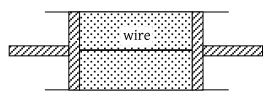When an ideal diatomic gas is heated at constant pressure, the fraction of the heat energy supplied which increases the internal energy of the gas, is -

(1) $\frac{2}{5}$

(2) $\frac{3}{5}$

(3) $\frac{3}{7}$

(4) $\frac{5}{7}$

Concept Questions :-

Molar Specific heat
High Yielding Test Series + Question Bank - NEET 2020

Difficulty Level:

A monoatomic ideal gas, initially at temperature T1, is enclosed in a cylinder fitted with a frictionless piston. The gas is allowed to expand adiabatically to a temperature T2 by releasing the piston suddenly. If L1 and L2 are the lengths of the gas column before and after expansion respectively, then T1/ T2 is given by -

(1) ${\left(\frac{{L}_{1}}{{L}_{2}}\right)}^{2/3}$

(2) $\frac{{L}_{1}}{{L}_{2}}$

(3) $\frac{{L}_{2}}{{L}_{1}}$

(4) ${\left(\frac{{L}_{2}}{{L}_{1}}\right)}^{2/3}$

Concept Questions :-

Types of processes
High Yielding Test Series + Question Bank - NEET 2020

Difficulty Level:

A closed hollow insulated cylinder is filled with gas at 0°C and also contains an insulated piston of negligible weight and negligible thickness at the middle point. The gas on one side of the piston is heated to 100°C. If the piston moves 5 cm, the length of the hollow cylinder is -

(1) 13.65 cm

(2) 27.3 cm

(3) 38.6 cm

(4) 64.6 cm

Concept Questions :-

Types of processes
High Yielding Test Series + Question Bank - NEET 2020

Difficulty Level:

A mono atomic gas is supplied the heat Q very slowly keeping the pressure constant. The work done by the gas will be

(1) $\frac{2}{3}Q$

(2) $\frac{3}{5}Q$

(3) $\frac{2}{5}Q$

(4) $\frac{1}{5}Q$

Concept Questions :-

Molar Specific heat
High Yielding Test Series + Question Bank - NEET 2020

Difficulty Level:

A gas mixture consists of 2 moles of oxygen and 4 moles argon at temperature T. Neglecting all vibrational modes, the total internal energy of the system is

(1) 4 RT

(2) 15 RT

(3) 9 RT

(4) 11 RT

Concept Questions :-

Basic terms
High Yielding Test Series + Question Bank - NEET 2020

Difficulty Level:

An ideal gas expands isothermally from a volume V1 to V2 and then compressed to original volume V1 adiabatically. Initial pressure is P1 and final pressure is P3. The total work done is W. Then -

(1) ${P}_{3}>{P}_{1},\text{\hspace{0.17em}\hspace{0.17em}}W>0$

(2) ${P}_{3}<{P}_{1},\text{\hspace{0.17em}\hspace{0.17em}}W<0$

(3) ${P}_{3}>{P}_{1},\text{\hspace{0.17em}\hspace{0.17em}}W<0$

(4) ${P}_{3}={P}_{1},\text{\hspace{0.17em}\hspace{0.17em}}W=0$

Concept Questions :-

Work done by gas
High Yielding Test Series + Question Bank - NEET 2020

Difficulty Level:

Work done by a system under isothermal change from a volume V1 to V2 for a gas which obeys Vander Waal's equation $\left(V-\beta n\right)\text{\hspace{0.17em}}\left(P+\frac{\alpha {n}^{2}}{V}\right)=nRT$

(1) $nRT{\mathrm{log}}_{e}\left(\frac{{V}_{2}-n\beta }{{V}_{1}-n\beta }\right)+\alpha \text{\hspace{0.17em}}{n}^{2}\text{\hspace{0.17em}}\left(\frac{{V}_{1}-{V}_{2}}{{V}_{1}{V}_{2}}\right)$

(2) $nRT{\mathrm{log}}_{10}\left(\frac{{V}_{2}-\alpha \beta }{{V}_{1}-\alpha \beta }\right)+\alpha \text{\hspace{0.17em}}{n}^{2}\text{\hspace{0.17em}}\left(\frac{{V}_{1}-{V}_{2}}{{V}_{1}{V}_{2}}\right)$

(3) $nRT{\mathrm{log}}_{e}\left(\frac{{V}_{2}-n\alpha }{{V}_{1}-n\alpha }\right)+\beta \text{\hspace{0.17em}}{n}^{2}\text{\hspace{0.17em}}\left(\frac{{V}_{1}-{V}_{2}}{{V}_{1}{V}_{2}}\right)$

(4) $nRT{\mathrm{log}}_{e}\left(\frac{{V}_{1}-n\beta }{{V}_{2}-n\beta }\right)+\alpha \text{\hspace{0.17em}}{n}^{2}\text{\hspace{0.17em}}\left(\frac{{V}_{1}{V}_{2}}{{V}_{1}-{V}_{2}}\right)$

Concept Questions :-

Work done by gas
High Yielding Test Series + Question Bank - NEET 2020

Difficulty Level:

A cylindrical tube of uniform cross-sectional area A is fitted with two air tight frictionless pistons. The pistons are connected to each other by a metallic wire. Initially the pressure of the gas is P0 and temperature is T0, atmospheric pressure is also P0. Now the temperature of the gas is increased to 2T0, the tension in the wire will be(1) 2 P0A

(2) P0A

(3) $\frac{{P}_{0}A}{2}$

(4) 4 P0A

Concept Questions :-

Types of processes
High Yielding Test Series + Question Bank - NEET 2020

Difficulty Level:

The molar heat capacity in a process of a diatomic gas if it does a work of $\frac{Q}{4}$ when a heat of Q is supplied to it is -

(1) $\frac{2}{5}R$

(2) $\frac{5}{2}R$

(3) $\frac{10}{3}R$

(4) $\frac{6}{7}R$

Concept Questions :-

Molar Specific heat
High Yielding Test Series + Question Bank - NEET 2020

Difficulty Level:

An insulator container contains 4 moles of an ideal diatomic gas at temperature T. Heat Q is supplied to this gas, due to which 2 moles of the gas are dissociated into atoms but temperature of the gas remains constant. Then

(1) Q = 2RT

(2) Q = RT

(3) Q = 3RT

(4) Q = 4RT

Concept Questions :-

First law of thermodynamics